# MATH 251: Calculus 3, SET8

## 12: Vectors and the Geometry of Space

### 12.3: The Dot Product

These problems are done with the CAS. See Hand Solutions for details.

#### 1. [812/8]

The dot product of two vectors specified via components is computed.
a = sym([3 2 -1]), b = sym([4 0 5])
a =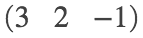b =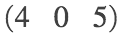a_dot_b = dot(a,b)
a_dot_b = 7

#### 2. [812/10]

The dot product of two vectors specified via magnitudes and included angle is computed.
length_of_a = 80, length_of_b = 50, theta = sym(3*pi/4)
length_of_a = 80
length_of_b = 50
theta =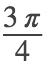a_dot_b = length_of_a * length_of_b * cos(theta)
a_dot_b =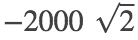#### 3. [812/12]

The dot products of vectors are computed using a figure in the statement of the problem.
length_of_u = sym(1), length_of_v = sym(1/sqrt(2)), length_of_w = 1
length_of_u = 1
length_of_v =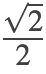length_of_w = 1
alpha = sym(pi/4), beta = sym(pi/2)
alpha =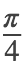beta =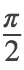u_dot_v = length_of_u * length_of_v * cos(alpha)
u_dot_v =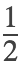u_dot_w = length_of_u * length_of_w * cos(beta)
u_dot_w = 0

#### 4. [813/22]

The angles of a triangle with specified vertices are computed.
A = [1 0 -1], B = [3 -2 0], C = [1 3 3]
A = 1×3
1 0 -1
B = 1×3
3 -2 0
C = 1×3
1 3 3
alpha = acosd(dot(B-A, C-A) / (norm(B-A) * norm(C-A)))
alpha = 97.6623
beta = acosd(dot(A-B, C-B) / (norm(A-B) * norm(C-B)))
beta = 53.5008
gamma = acosd(dot(A-C, B-C) / (norm(A-C) * norm(B-C)))
gamma = 28.8370
check = alpha + beta + gamma % Sum of angles of triangle equals 180 degrees.
check = 180

#### 5. [813/24]

Pairs of vectors are determined to be orthogonal (perpendicular), parallel, or neither.
% (a)
u = [-5 4 -2], v = [3 4 -1]
u = 1×3
-5 4 -2
v = 1×3
3 4 -1
u_dot_v = dot(u,v) % Since dot product is nonzero, the vectors are not perpendicular.
u_dot_v = 3
u_by_b = u./v % Since u is not a constant multiple of V, the vectors are not parallel.
u_by_b = 1×3
-1.6667 1.0000 2.0000
% Therefore, the vectors are neither perpendicular nor parallel.
% (b)
u = [9 -6 3], v = [-6 4 -2]
u = 1×3
9 -6 3
v = 1×3
-6 4 -2
u_by_v = u./v % Since u = 1.5*v is a constant multiple of v, u and v are parallel.
u_by_v = 1×3
-1.5000 -1.5000 -1.5000
% (c)
syms c
u = [c c c], v = [c 0 -c]
u =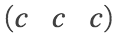v =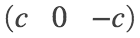u_dot_v = dot(u,v) % Since dot product is zero, the vectors are perpendicular.
u_dot_v = 0

#### 6. [813/28]

Two unit vectors are found that make an angle of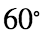with a specified vector.
v = sym([3 4]) % the specified vector
v =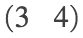u = v / norm(v) % a unit vector in the direction of the specified vector
u =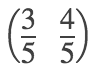alpha = atan(sym(4/3))
alpha =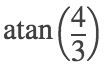u_polar = [1 alpha] % polar representation of u
u_polar =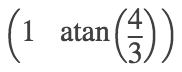w = [1 alpha+pi/3] % Rotate u clockwise 60 degrees (pi/3 radians)
w =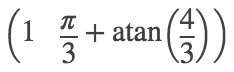z = [1 alpha-pi/3] % Rotate u counterclockwise 60 degrees (pi/3 radians)
z =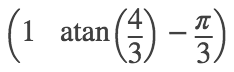w_rect = double(1*[cos(w(2)), sin(w(2))]) % w in rectangular components (approx)
w_rect = 1×2
-0.3928 0.9196
z_rect = double(1*[cos(z(2)), sin(z(2))]) % z in rectangular components (approx)
z_rect = 1×2
0.9928 -0.1196
w_angle = atan2d(w_rect(2), w_rect(1)) % angle in deg for w w.r.t. positive x-axis (approx)
w_angle = 113.1301
z_angle = atan2d(z_rect(2), z_rect(1)) % angle in deg for z w.r.t. positive x-axis (approx)
z_angle = -6.8699
length_of_w = norm(w_rect) % unit vector
length_of_w = 1
length_of_z = norm(z_rect) % unit vector
length_of_z = 1
% Illustrate
figure
quiver(0,0, 3/5,4/5, 'b', 'AutoScale', 'off'); grid on; hold on
quiver(0,0, w_rect(1),w_rect(2), 'r', 'AutoScale', 'off')
quiver(0,0, z_rect(1),z_rect(2), 'm', 'AutoScale', 'off')
plot([-1 1], [0 0], 'k')
plot([0 0], [-0.5 1], 'k')
axis equal; axis([-0.5 1 -0.5 1])
xlabel('x'); ylabel('y')
title('SET8, 813/28')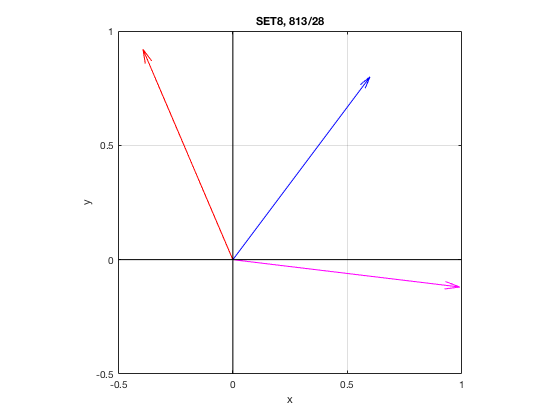#### 7. [813/30]

Write the lines as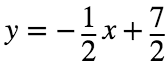and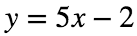. The angles these lines make with the positve x-axis are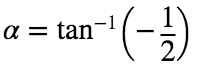and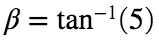, respectively. So the obtuse angle between the lines is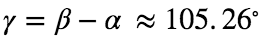. The acute angle between the lines is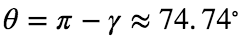.
alpha = atan2d(-1,2)
alpha = -26.5651
beta = atan2d(5,1)
beta = 78.6901
gamma = beta - alpha
gamma = 105.2551
theta = 180 - gamma
theta = 74.7449
%
syms x
figure
fplot(-1/2*x + 7/2); grid on; hold on
fplot(5*x - 2)
plot([-2 2], [0 0], 'k')
plot([0 0], [-5 5], 'k')
plot(1, 3, 'mo', 'MarkerFaceColor', 'm')
axis equal; axis([-2 2 -2 5])
xlabel('x'); ylabel('y')
title('SET8, 813/30')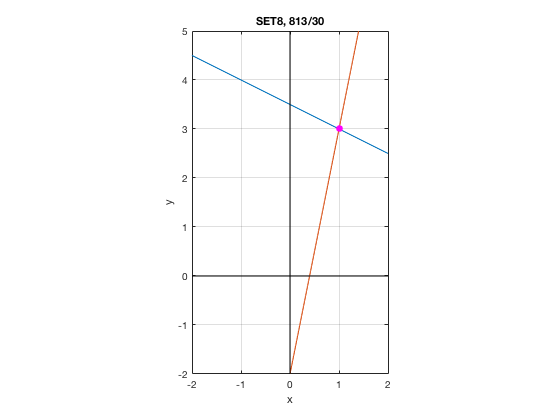#### 8. [813/32]

The acute angle between two curves at their point of intersection is computed.
syms x y
f(x) = sin(x), g(x) = cos(x)
f(x) =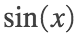g(x) =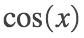Df(x) = diff(f(x), x), Dg(x) = diff(g(x), x)
Df(x) =Dg(x) =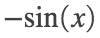xi = solve(f(x) == g(x), x)
xi =mf = Df(xi), mg = Dg(xi)
mf =mg =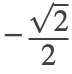theta = double(rad2deg(atan(mf) - atan(mg))) % angle betwee curves in degrees (approx)
theta = 70.5288
Lf(x) = expand(f(xi) + mf*(x - xi))
Lf(x) =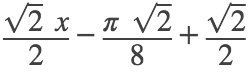Lg(x) = expand(g(xi) + mg*(x - xi))
Lg(x) =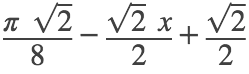P = [xi, f(xi)]
P =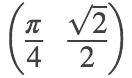figure
fplot(f(x), [0 pi/2], 'LineWidth', 2); grid on; hold on
fplot(g(x), [0 pi/2], 'LineWidth', 2); grid on; hold on
fplot(Lf(x), [0 pi/2], 'b--');
fplot(Lg(x), [0 pi/2], 'r--');
plot(P(1), P(2), 'mo', 'MarkerFaceColor', 'm', 'MarkerSize', 10)
axis equal; axis([0 pi/2 0 1.5])
xlabel('x'); ylabel('y')
title('SET8, 813/32')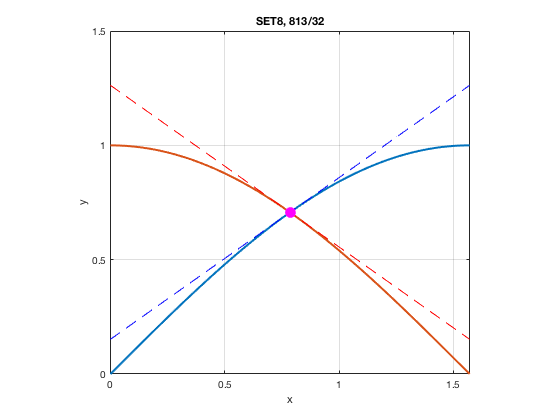#### 9. [813/44]

Scalar and vector projections are computed.
a = sym([1 2 3]), b = sym([5 0 -1])
a =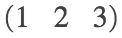b =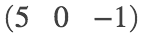comp_a_b = dot(a,b) / norm(a)
comp_a_b =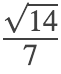comp_a_b_appx = double(comp_a_b)
comp_a_b_appx = 0.5345
proj_a_b = comp_a_b * a/norm(a)
proj_a_b =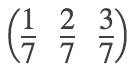proj_a_b_appx = double(proj_a_b)
proj_a_b_appx = 1×3
0.1429 0.2857 0.4286

#### 10. [813/52]

A boat sails south with the help of a wind blowing in the direction S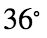E with magnitude 400 lb. Find the work done by the wind as the boat moves 120 ft.
Work is the dot product of force with displacement F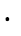D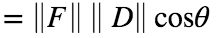.
mag_F = 400; mag_D = 120; theta = 36 % degrees
theta = 36
format bank
work = mag_F * mag_D * cosd(36) % work in ft-lb
work = 38832.82
format short